# ILP Part 30 — Sudoku

This is the thirtieth part of the ILP series. For your convenience you can find other parts in the table of contents in Part 1 – Boolean algebra

Hello! Today we are going to solve Sudoku using ILP.

# Description

In Sudoku we need to fill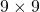grid with numbers. In every column, in every row, and in nine smaller subgrids we can use every number fromtoexactly once. We usually start with few fields filled and we need to find the rest. See the picture below (from wikipedia):In this post we will use basic set operations a lot so make sure that you understand them.

# Variables

Let’s start with variables for the problem. We usually use binary variables to indicate decision, however, this time we will use integer variables in range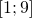to represent the solutions. It is quite intuitive: after solving the problem we obtain the result by checking the value of variable for given board field. So we define the following variables: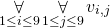We also make sure that they are in the correct range: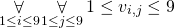Let’s now define constraints.

# Constraints

This part is very easy. We already know how to define “all different” constraint, so all we need to do is to add constraints for every row, every column, and every subgrid: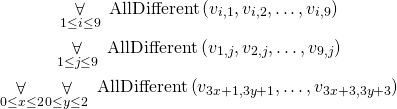Output:

# Summary

As we can see, our toolkit for building ILP formulas is quite powerfull and we are able to solve Sudoku almost instantly just by translating rules of the game (nine different numbers in each column, nine different numbers in each row, nine different numbers in each subgrid) to mathematical operators.